# Thread: How to match wideband output to {B3601}

1. ##How to match wideband output to {B3601}

WideBand Oxygen Sensors measure the amount of residual oxygen in the exhaust gas and convert it to Lambda value.
Some WBO2 controllers enable you to convert the Lambda values into AFR based on user defined Stoichiometric AFR.
Most do not but give you the expression that converts Lambda into AFR from their defined Stoichiometric AFR.
This pre-defined expression will not exactly match the Stoich AFR value of {B3601} and is usually 14.7:1

If using AFR for a reference it is important that the AFR value of {B3601} match the AFR output slope of the WBO2.
If not the BEN factor multiplier will be incorrect making it unreliable for tuning the VE and MAF.

Example #1:
{B3601}=14.68 & {CALC.AFR_NGK} WBO2 expression of Lambda1=14.57 (V*1.4)+9
14.57/14.68=0.99

This is a small amount of error but there should not be any.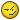Not a big deal but if {B3601} differs significantly from the AFR slope,
BEN will be even further away from what it should be.

Example #2:
{B3601}=14.13 & {CALC.AFR_PLX} WBO2 expression of Lambda1=14.7 (V*2)+10
14.13/14.7=0.96

Here you can see BEN would remove 4% when it should not.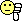To fix this either change {B3601} to match the WBO2 slope as close as possible or
make a new expression using the slope intercept formula of y=(m*x)+b
with the Lambda & voltage range of your WBO2 and the AFR value of {B3601}
{B3601}*maximum Lambda=a
{B3601}*minimum Lambda=b (intercept)
(a-b)/voltage range=m (slope)
x=volts from WBO2
y=AFR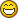This spreadsheet will do the work for you.>>>>WBO2Expressions.xls<<<<

Input your WBO2's Lambda & Voltage range in the blue & red cells of column B then
paste the value of {B3601} into cell B13.
The AFR range is not necessary.
Creating a multiplier from WBO2 Lambda and {GM.EQUIVRATIO} is another solution.
"({Lambda}*{GM.EQUIVRATIO})"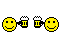Reply With Quote

2. ##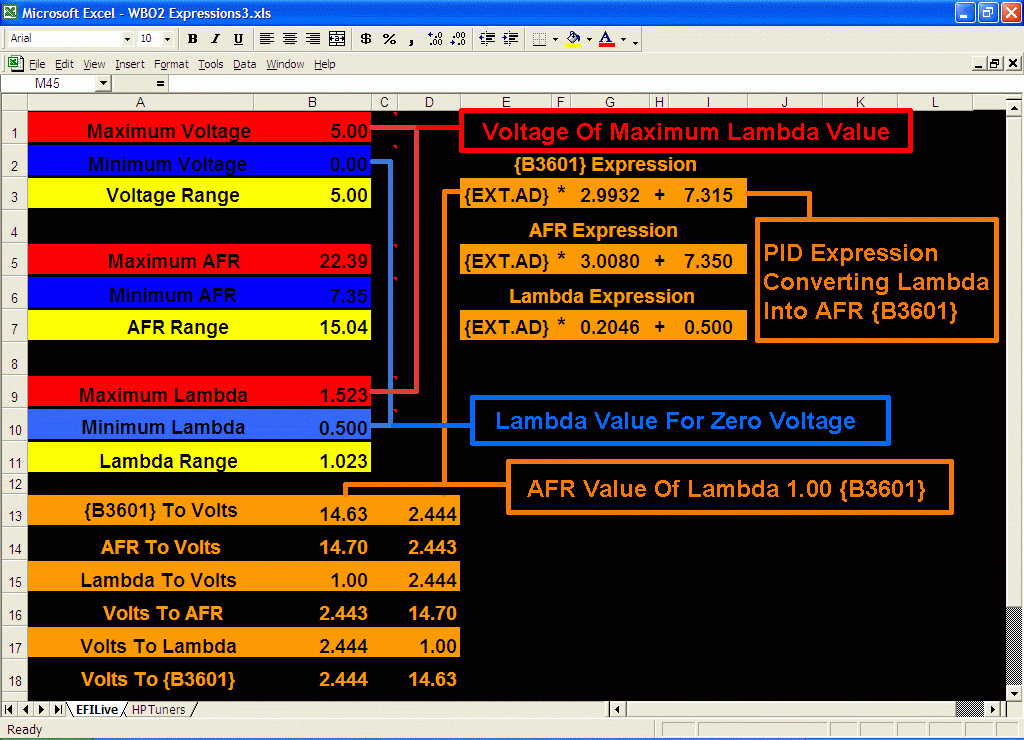Reply With Quote

####Posting Permissions

• You may not post new threads
• You may not post replies
• You may not post attachments
• You may not edit your posts
•